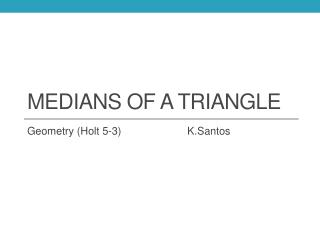DownloadDownload PresentationMedians of a Triangle

# Medians of a Triangle

Download Presentation## Medians of a Triangle

- - - - - - - - - - - - - - - - - - - - - - - - - - - E N D - - - - - - - - - - - - - - - - - - - - - - - - - - -
##### Presentation Transcript

1. Medians of a Triangle Geometry (Holt 5-3) K.Santos

2. Median of a triangle Median of a triangle is a segment whose endpoints are a vertex of the triangle and the midpoint of the opposite side.

3. Centroid of a triangle Centroid of a triangle is the point of concurrency of the medians of the triangle.

4. Centroid Theorem (5-3-1) The centroid of a triangle is located of the distance form each vertex to the midpoint of the opposite side. B X Y P A C Z AP = AY BP = BZ CP = CX YP = AY ZP = BZ XP = CX

5. Centroid 2 6 1 3 2 + 1 = 3 6 + 3 = 9 Longer segment reduced is Shorter segment reduced is

6. Centroid Example B In ABC,G is the centroid. AF = 9 and GE = 2.4. Find each length. E F G Find AG AG = AF A D C AG = (9) AG = 6 Find CE GC = CE and GE = CE 2.4 = CE 7.2 = CE (multiplied by 3)